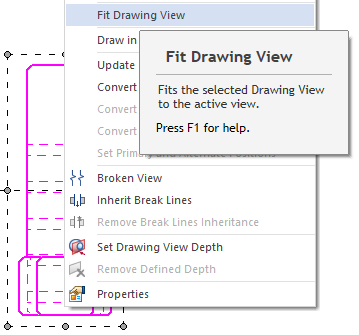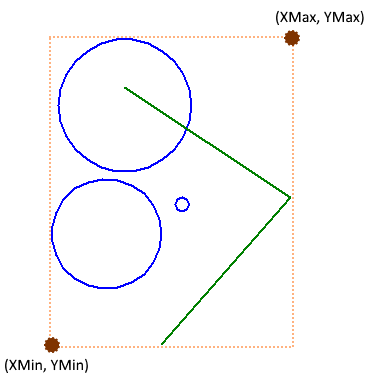# Conversions and Transformations using the Solid Edge API – Part 2

Continuing from the previous part that discussed the UnitsOfMeasure object, this part explains the conversion of sheet coordinates to view coordinates.

This series of articles discusses the following:

1. Document Unit Conversion – ParseUnit and Format Unit methods of the UnitsOfMeasure object.

2. View Coordinate Conversion – ViewToSheet and SheetToView methods of the DrawingView object.

3. 2D to 3D Conversion – Convert2DCoordinate method of the Profile object.

4. 3D to 2D Transformations – ModelToView method of the DrawingView object.

5. Assembly Transformations – GetMatrix and PutMatrix methods of the Occurrence object.

View Coordinates Conversion

When you right-click a drawing view in a Draft sheet, one of the options is to zoom fit to the Drawing ViewBut other simpler drawing objects like lines and circles do not have this feature. Let’s implement it.

The coordinates of the bounding box or extents of an object can be determined by the Range Method.

```Public Sub Range( _
ByRef XMin As Double, _
ByRef YMin As Double, _
ByRef XMax As Double, _
ByRef YMax As Double _
)```

And depicted as below:The image above shows the range of a bunch of objects for which a direct API does not exist.

The function below determines the combined Range for a set of selected objects in Solid Edge Draft:

First check if the user has selected at least one drawing object:

```Sub Main()
Dim seApp As SolidEdgeFramework.Application =   System.Runtime.InteropServices.Marshal.GetActiveObject(“solidedge.application”)
Dim seDoc As SolidEdgeDraft.DraftDocument = seApp.ActiveDocument
Dim seSheet As SolidEdgeDraft.Sheet = seDoc.ActiveSheet
Dim sSet As SelectSet = seDoc.SelectSet
If sSet.Count = 0 Then
MsgBox(“select atleast one object.”)
End
End If```

Next, take the first object in the select set as a seed object and get its range:

oSel.Item(1).Range(XMin, YMin, XMax, YMax)

Loop through all the objects in the select set and compare their extents with the seed object to determine which one has a greater extent:

```For i = 1 To oSel.Count
oSel.Item(i).Range(X1, Y1, X2, Y2)
If X1 < XMin Then
XMin = X1
End If
If Y1 < YMin Then
YMin = Y1
End If
If X2 > XMax Then
XMax = X1
End If
If Y2 > YMax Then
YMax = Y2
End If
Next```

This finds the extents or bounding box of the objects combined.

These values of the bounding box are in terms of the document units and the sheet coordinates increase in the North-East direction i.e. from Lower-Left corner to Upper-Right corner.

For zooming the view to these coordinates, they need to be converted to the window or view coordinates who increase in the South-East direction meaning from Top-Left to the Bottom-Right corner as shown below:Convert the values obtained by the Range method to window coordinates using the ModelToWindow method:

```oSheetWindow = oApp.ActiveWindow
oSheetWindow.ModelToWindow(XMin, YMin, XWinMin, YWinMin)
oSheetWindow.ModelToWindow(XMax, YMax, XWinMax, YWinMax)```

Use these converted coordinates to zoom in using the ZoomArea method:

```If XWin1 = XWin2 Then
XWin2 *= 1.1
End If
If YWin1 = YWin2 Then
YWin2 *= 1.1
End If
oSheetWindow.ZoomArea(XWin1, YWin1, XWin2, YWin2)```

Add some extra checks before zooming to the area for vertical or horizontal lines since their coordinates could be the same and an area or window is not formed.

Now you have a brand new command that zooms in an area formed by the extents of a bunch of selected object in Solid Edge Draft.

In the next part of this article, I will show you how to perform a 3-step conversion of the center point coordinates of a hole in a part model all way to its coordinate in the Drawing Sheet in Draft.

If you have any questions related to this article, kindly post them on the Solid Edge Developer forum.